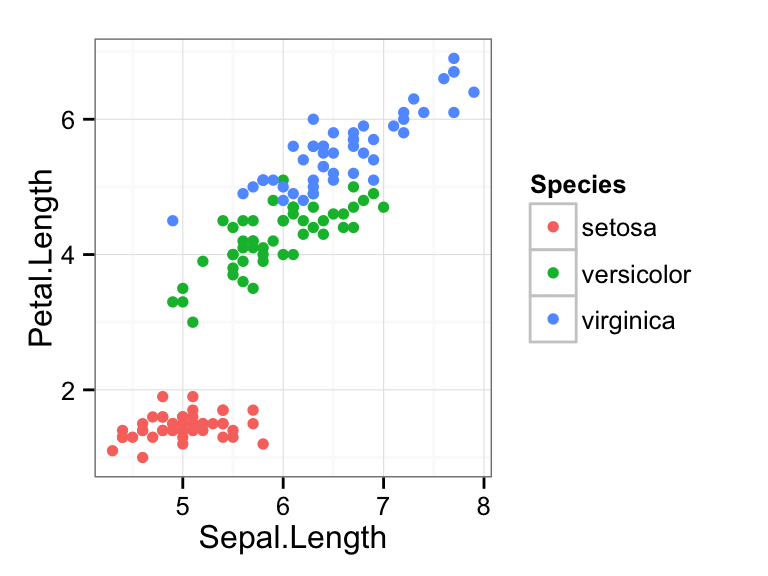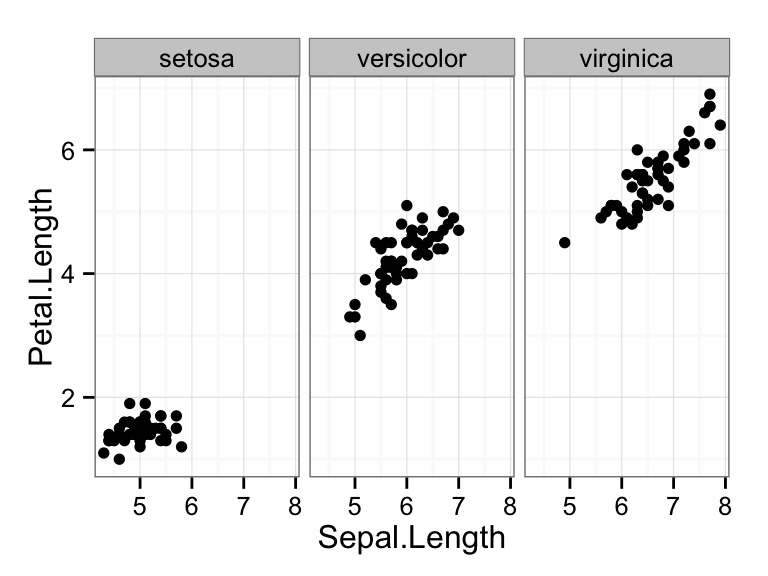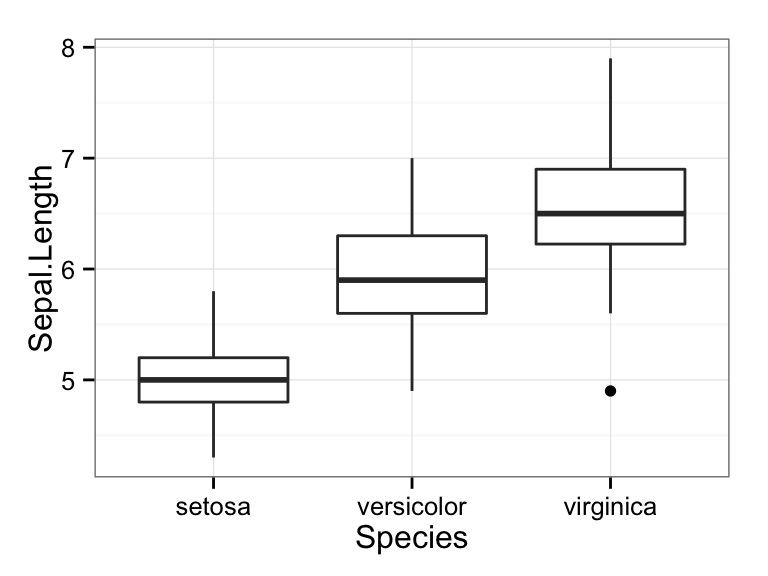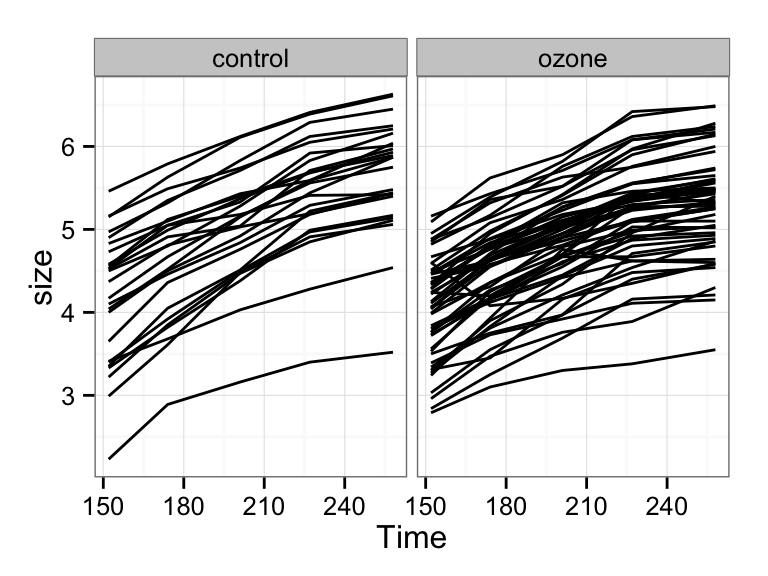## In-class worksheet, class 16

We will be using the R package ggplot2 for all plots. To use it, we first need to load it:

``library(ggplot2)``

The default theme of ggplot2 is not the most beautiful. This code switches to a more pleasant theme:

``theme_set(theme_bw())``

## 1. Plotting the iris data set.

For this exercise we are using the `iris` data set available in R. This data set gives the measurements in centimeters of the variables sepal length and width and petal length and width, respectively, for 50 flowers from each of 3 species of iris. The species are Iris setosa, versicolor, and virginica:

``head(iris)``
``````##   Sepal.Length Sepal.Width Petal.Length Petal.Width Species
## 1          5.1         3.5          1.4         0.2  setosa
## 2          4.9         3.0          1.4         0.2  setosa
## 3          4.7         3.2          1.3         0.2  setosa
## 4          4.6         3.1          1.5         0.2  setosa
## 5          5.0         3.6          1.4         0.2  setosa
## 6          5.4         3.9          1.7         0.4  setosa``````

Make a scatter plot of petal length vs. sepal length for the three species. Make a single plot that shows the data for all three species at once, in different colors. Then do the same plot but facet by species instead of coloring.

``ggplot(iris, aes(x=Sepal.Length, y=Petal.Length, color=Species)) + geom_point()````ggplot(iris, aes(x=Sepal.Length, y=Petal.Length)) + geom_point() + facet_wrap(~Species)``Now see if you can make side-by-side boxplots of sepal lengths for the three species of iris. The geom you need to use is `geom_boxplot()`. See if you can guess the correct aesthetic mapping.

``ggplot(iris, aes(y=Sepal.Length, x=Species)) + geom_boxplot() ``## 2. Plotting tree-growth data.

The data set `Sitka` from the MASS package contains repeated measurements of tree size for 79 Sitka spruce trees, which were grown either in ozone-enriched chambers or under control conditions.

``````library(MASS) # we need to load the MASS library to have access to this dataset
``````##   size Time tree treat
## 1 4.51  152    1 ozone
## 2 4.98  174    1 ozone
## 3 5.41  201    1 ozone
## 4 5.90  227    1 ozone
## 5 6.15  258    1 ozone
## 6 4.24  152    2 ozone``````

Make line plots of tree size vs. time, for each tree, faceted by treatment. First, use the same color for all lines.

``ggplot(Sitka, aes(x=Time, y=size, group=tree)) + geom_line() + facet_wrap(~treat)``Then, color by tree.

``ggplot(Sitka, aes(x=Time, y=size, color=tree, group=tree)) + geom_line() + facet_wrap(~treat)``